# Decoding a grid square

I saw a reference last night to the grid square EL29fx and wanted to figure out where that is. There are many programs that will do this for you, but I wanted to do it by hand.

I wrote about how grid squares work a year ago, but I was rusty on the details, so this morning I went back and reread my earlier post.

## Parsing a locator

The system used to create grid squares in amateur radio is the Maidenhead Locator System. Last year I wrote about why the system works the way it does. Here I will recap how the system works but refer you to the earlier post for the motivation behind the design choices.

First of all, the Maidenhead system interleaves the longitude and latitude information. So in the square EL29fx, the longitude information is (E, 2, f) and the latitude information is (L, 9, x). The first two characters, EL, gives a rough location, a “field.” Then the next two characters, 29, refine the location to a “square.” The last two, fx, narrow the square down to a “subsquare.”

## Finding the field

The first character in the grid square specifies a range of longitude east of the International Date Line . Each letter represents a range of 20° longitude: A for 0 to 20° longitude, B for 20° to 40°, etc. So E represents 80° to 100° longitude east of the antemeridian or 260° to 280° east of the prime meridian.

The second character in the grid square represents latitude north of the south pole in increments of 10°. So L represents 110° to 120° north of the south pole, or 20° to 30° north of the equator.

Together, EL tells us we’re looking at some place 260° to 280° E and 20° to 30° N, i.e. somewhere in Mexico or the US Gulf Coast.

## Finding the square

The two digits in the middle of a grid square divide the field into 10 parts in both directions. Since a field is 20° wide and 10° tall, the first digit represents multiples of 2° longitude and the second digit represent multiples of 1° latitude.

So if we look at EL29, we know our location is between 264° and 266° E and between 29° and 30° N, which is somewhere around Houston, Texas

## Finding the subsquare

A subsquare divides a square into 24 parts in each direction. So a subsquare is 5 minutes of longitude wide and 2.5 minutes of latitude high.

The f in EL29fx refines the longitude is 264° 25′ E (95° 35′ W) and the x refines the latitude is 29° 55′ N. This narrows the location to somewhere in northwest Houston.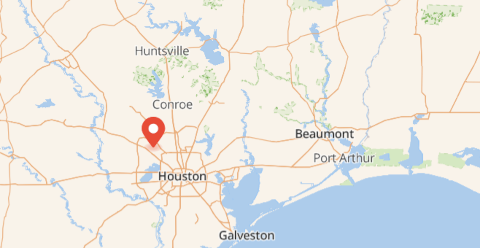There’s no significance to the case of the letters other than as a reminder that the first and second pair of letters are interpreted differently.

 Technically, degrees east of 180th meridian, a.k.a. the antemeridian, which mostly coincides with the International Date Line. The latter zigzags a little to avoid splitting island groups.

# Oscillations in RLC circuits

Electrical and mechanical oscillations satisfy analogous equations. This is the basis of using the word “analog” in electronics. You could study a mechanical system by building an analogous circuit and measuring that circuit in a lab.

## Mass, dashpot, spring

Years ago I wrote a series of four posts about mechanical vibrations:

Everything in these posts maps over to electrical vibrations with a change of notation.

That series looked at the differential equationwhere m is mass, γ is damping from a dashpot, and k is the stiffness of a spring.

## Inductor, resistor, capacitor

Now we replace our mass, dashpot, and spring with an inductor, resistor, and capacitor.

Imagine a circuit with an L henry inductor, and R ohm resistor, and a C farad capacitor in series. Let Q(t) be the charge in coulombs over time and let E(t) be an applied voltage, i.e. an AC power source.

### Charge formulation

One can use Kirchhoff’s law to deriveHere we have the correspondencesSo charge is analogous to position, inductance is analogous to mass, resistance is analogous to damping, and capacitance is analogous to the reciprocal of stiffness.

The reciprocal of capacitance is called elastance, so we can say elastance is proportional to stiffness.

### Current formulation

It’s more common to see the differential equation above written in terms of current I.If we take the derivative of both sides ofwe get## Natural frequency

With mechanical vibrations, as shown here, the natural frequency isand with electrical oscillations this becomesWhen a mechanical or electrical system is driven by sinusoidal forcing function, the system eventually settles down to a solution that is proportional to a phase shift of the driving function.

To be more explicit, the solution to the differential equationhas a transient component that decays exponentially and a steady state component proportional to cos(ωt-φ). The same is true of the equationThe proportionality constant is conventionally denoted 1/Δ and so the steady state solution isfor the mechanical case andfor the electrical case.

The constant Δ satisfiesfor the mechanical system andfor the electrical system.

When the damping force γ or the resistance R is small, then the maximum amplitude occurs when the driving frequency ω is near the natural frequency ω0.

More on damped, driven oscillations here.

# How is portable AM radio possible?

The length of antenna you need to receive a radio signal is proportional to the signal’s wavelength, typically 1/2 or 1/4 of the wavelength. Cell phones operate at gigahertz frequencies, and so the antennas are small enough to hide inside the phone.

But AM radio stations operate at much lower frequencies. For example, there’s a local station, KPRC, that broadcasts at 950 kHz, roughly one megahertz. That means the wavelength of their carrier is around 300 meters. An antenna as long as a quarter of a wavelength would be roughly as long as a football field, and yet people listen to AM on portable radios. How is that possible?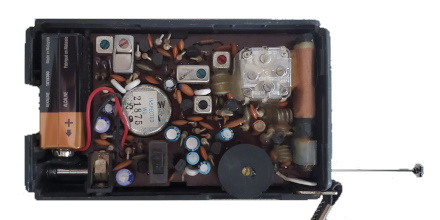There are two things going on. First, transmitting is very different than receiving in terms of power, and hence in terms of the need for efficiency. People are not transmitting AM signals from portable radios.

Second, the electrical length of an antenna can be longer than its physical length, i.e. an antenna can function as if it were longer than it actually is. When you tune into a radio station, you’re not physically making your antenna longer or shorter, but you’re adjusting electronic components that make it behave as if you were making it longer or shorter. In the case of an AM radio, the electrical length is orders of magnitude more than the physical length. Electrical length and physical length are closer together for transmitting antennas.

Here’s what a friend of mine, Rick Troth, said when I asked him about AM antennas.

If you pop open the case of a portable AM radio, you’ll see a “loop stick”. That’s the AM antenna. (FM broadcast on most portables uses a telescoping antenna.) The loop is tuned by two things: a ferrite core and the tuning capacitor. The core makes the coiled wiring of the antenna resonate close to AM broadcast frequencies. The “multi gang” variable capacitor coupled with the coil forms an LC circuit, for finer tuning. (Other capacitors in the “gang” tune other parts of the radio.) The loop is small, but is tuned for frequencies from 530KHz to 1.7MHz.

Loops are not new. When I was a kid, I took apart so many radios. Most of the older (tube type, and AM only) radios had a loop inside the back panel. Quite different from the loop stick, but similar electrical properties.

Car antennas don’t match the wavelengths for AM broadcast. Never have. That’s a case where matching matters less for receivers. (Probably matters more for satellite frequencies because they’re so weak.) Car antennas, whether whip from decades ago or embedded in the glass, probably match FM broadcast. (About 28 inches per side of a dipole, or a 28 inch quarter wave vertical.) But again, it does matter a little less for receive than for transmit.

In the photo above, courtesy Rick, the AM antenna is the copper coil on the far right. The telescoping antenna outside the case extends to be much longer physically than the AM antenna, even though AM radio waves are two orders of magnitude longer than FM radio waves.

# Multiple Frequency Shift Keying

A few days ago I wrote about Frequency Shift Keying (FSK), a way to encode digital data in an analog signal using two frequencies. The extension to multiple frequencies is called, unsurprisingly, Multiple Frequency Shift Keying (MFSK). What is surprising is how MFSK sounds.

When I first heard MFSK I immediately recognized it as an old science fiction sound effect. I believe it was used in the original Star Trek series and other shows. The sound is in once sense very unusual, which is why it was chosen as a sound effect. But in another sense it’s familiar, precisely because it has been used as a sound effect.

Each FSK pulse has two possible states and so carries one bit of information. Each MFSK pulse has 2n possible states and so carries n bits of information. In practice n is often 3, 4, or 5.

## Why does it sound strange?

An MFSK signal will jump between the possible frequencies in no apparent; if the data is compressed before encoding, the sequence of frequencies will sound random. But random notes on a piano don’t sound like science fiction sound effects. The frequencies account for most of the strangeness.

MFSK divides its allowed bandwidth into uniform frequency intervals. For example, a 500 Hz bandwidth might be divided into 32 frequencies, each 500/32 Hz apart. The tones sound strange because they are uniformly on a linear scale, whereas we’re used to hearing notes uniformly spaced on a logarithmic scale. (More on that here.)

In a standard 12-note chromatic scale, the ratios between consecutive frequencies is constant, each frequency being about 6% larger than the previous one. More precisely, the ratio between consecutive frequencies equals the 12th root of 2. So if you take the logarithm in base 2, the distance between each of the notes is 1/12.

In MFSK the difference between consecutive frequencies is constant, not the ratio. This means the higher frequencies will sound closer together because their ratios are closer together.

## Pulse shaping

As I discussed in the post on FSK, abrupt frequency changes would cause a signal to use an awful lot of bandwidth. The same is true of MFSK, and as before the solution is to taper the pulses to zero on the ends by multiplying each pulse by a windowing function. The FSK post shows how much bandwidth this saves.

When I created the audio files below, at first I didn’t apply pulse shaping. I knew it was important to signal processing, but I didn’t realize how important it is to the sound: you can hear the difference, especially when two consecutive frequencies are the same.

## Audio files

The following files are a 5-bit encoding. They encode random numbers k from 0 to 31 as frequencies of 1000 + 1000k/32 Hz.

Here’s what a random sample sounds like at 32 baud (32 frequency changes per second) with pulse shaping.

32 baud

Here’s the same data a little slower, at 16 baud.

And here is is even slower, at 8 baud.

## Examples

Here’s one example I found: “Sequence 2” from
this page of sound effects sounds like a combination of teletype noise and MFSK. The G7 computer sounds like MFSK too.

# Frequency shift keying (FSK) spectrum

This post will look encoding digital data as an analog signal using frequency shift keying (FSK), first directly and then with windowing. We’ll look at the spectrum of the encoded signal and show that basic FSK uses much less bandwidth than direct encoding, but more bandwidth than FSK with windowing.

## Square waves

The most natural way to encode binary data as an analog signal would be represent 0s and 1s by a sequence of pulses that take on the values 0 and 1.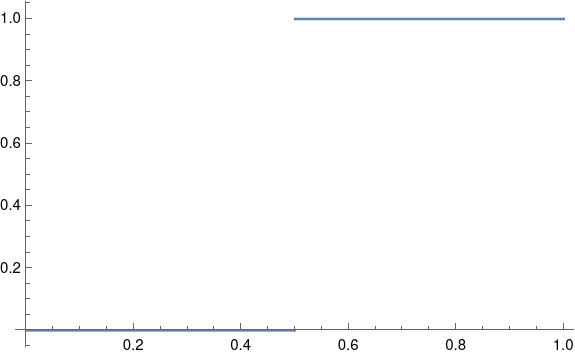A problem with this approach is that it would require a lot of bandwidth.

In theory a square wave has infinite bandwidth: its Fourier series has an infinite number of non-zero coefficients. In practice, the bandwidth of a signal is determined by how many Fourier coefficients it has above some threshold. The threshold would depend on context, but let’s say we ignore Fourier components with amplitude smaller than 0.001.

As I wrote about here, the nth Fourier sine series coefficients for a square wave is equal to 4/nπ for odd n. This means we would need on the order of 1,000 terms before the coefficients drop below our threshold.

## Frequency shift keying

The rate of convergence of the Fourier series for a function f depends on the smoothness of f. Discontinuities, like the jump in a square wave, correspond to slow convergence, i.e. high bandwidth. We can save bandwidth by encoding our data with smoother functions.

So instead of jumping from 0 to 1, we’ll encode a 0 as a signal of one frequency and a 1 as a signal with another frequency. By changing the frequency after some whole number of periods, the resulting function will be continuous, and so will have smaller bandwidth.

Suppose we have a one second signal f(t) that is made of half a second of a 4 Hz signal and half a second of a 6 Hz signal, possibly encoding a 0 followed by a 1.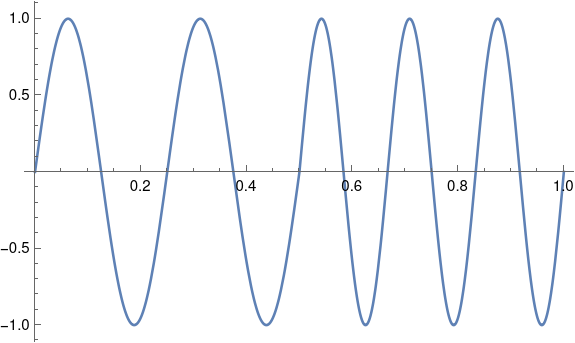What would the Fourier coefficients look like? If we just had a 4 Hz sine wave, the Fourier series would have only one component: the signal itself at 4 Hz. If we just had a 6 Hz sine wave, the only Fourier component would again be the signal itself. There would be no sine components at other frequencies, and no cosine components.

But our signal patched together by concatenating 4 Hz and 6 Hz signals has non-zero cosine terms for every odd n, and these coefficients decay like O(1/n²).

Our Fourier series is

f(t) = 0.25 sin 8πt + 0.25 sin 12πt + 0.0303 cos 2πt + 0.1112 cos 6πt – 0.3151 cos 12πt + 0.1083 cos 10πt + …

We need to go out to 141 Hz before the coefficients drop below 0.001. That’s a lot of coefficients, but it’s an order of magnitude fewer coefficients than we’d need for a square wave.

## Pulse shaping

Although our function f is continuous, it is not differentiable. The left-hand derivative at 1/2 is 8π and the right-hand derivative is 12π. If we could replace f with a related function that is differentiable at 1/2, presumably the signal would require less bandwidth.

We could do this by multiplying both halves of our signal by a windowing function. This is called pulse shaping because instead of a simple sine wave, we change the shape of the wave, tapering it at the ends.

Let’s using a cosine window because that’ll be easy; in practice you’d probably use a different window .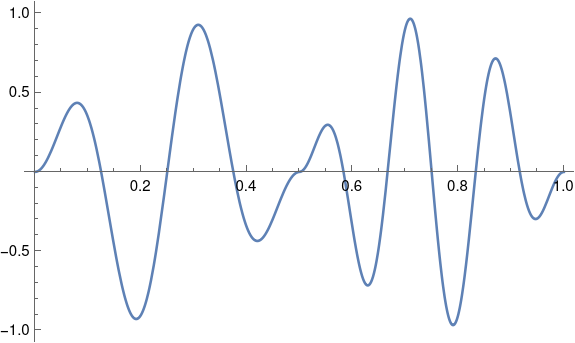Now our function is differentiable at 1/2, and its Fourier series converges more quickly. Now we can disregard components above 40 Hz. With a smoother windowing function the windowed function would have more derivatives and we could disregard more of the high frequencies.

## Related posts

 This kind of window is called a cosine window because you multiply your signal by one lobe of a cosine function, with the peak in the middle of the signal. Since we’re doing this over [0. 1/2] and again over [1/2, 1], we’re actually multiplying by |sin 2πt|.

The radio spectrum is conventionally  divided into frequency bands that seem arbitrary at first glance. For example, VHF runs from 30 to 300 MHz. All the frequency band boundaries are 3 times a power of 10.

Why all the 3’s? Two reasons: 3 is roughly the square root of 10, and the speed of light is 300,000 km/s. The former matters when you think in terms of frequencies, and the latter matters when you think in terms of wavelengths.

## Frequencies

The frequency bands are centered (on a log scale) on powers of 10. The band centered at 10n runs from

3 × 10n-1 Hz

to

3 × 10n Hz.

Since 100.5 ≈ 3, this means that the log base 10 of the frequency band runs from roughly n – 1/2 to n + 1/2.

    |--------+-------+-----------------------------|
| Center | Short | Long                        |
|--------+-------+-----------------------------|
|  10^0  | TLF   | Tremendously Low Frequency  |
|  10^1  | ELF   | Extremely Low Frequency     |
|  10^2  | SLF   | Super Low Frequency         |
|  10^3  | ULF   | Ultra Low Frequency         |
|  10^4  | VLF   | Very Low Frequency          |
|  10^5  |  LF   | Low Frequency               |
|  10^6  |  MF   | Medium Frequeny             |
|  10^7  |  HF   | High Frequency              |
|  10^8  | VHF   | Very High Frequency         |
|  10^9  | UHF   | Ultra High Frequency        |
|  10^10 | SHF   | Super High Frequency        |
|  10^11 | EHF   | Extremely High Frequency    |
|  10^12 | THF   | Tremendously High Frequency |
|--------+-------+-----------------------------|


The table is easier to remember if you read it from the middle because it’s symmetric about 1 MHz.

From MF you either go up to HF or down to LF. Above HF is VHF and below LF is VLF. Above VHF is UHF, and below VLF and ULF. And so on.

The following bit of Python code computes the name of a frequency’s band following the discussion above.

    from math import log10

def frequency_band(freq):
if decade in [5, 6, 7]:
else:
name += "H" if decade > 6 else "L"
return name + "F"


## Wavelengths

A radio wave with frequency f has wavelength c/f. As noted above, The band centered at 10n runs from 3 × 10n-1 to 3 × 10n. This means the wavelengths vary from

3 × 108 m / 3 × 10n

to

3 × 108 m / 3 × 10n-1

and so the wavelength is between 108-n and 109-n meters.

To change the code above take wavelength as an argument, calculate the decade as

    decade = 8 - int(log10(wavelength))

Note that casting to integer truncates floats, taking the floor before changing the type.

## Related posts

 Specifically, these are the conventions established by the International Telecommunications Union (ITU).

# Building high frequencies out of low frequencies

If you have sines and cosines of some fundamental frequency, and you’re able to take products and sums, then you can construct sines and cosines at any multiple of the fundamental frequency.

Here’s a proof.Taking real parts gives us cos nθ in the first equation and the even terms of the sum in the last equation.

Taking imaginary parts gives us sin nθ in the first equation and the odd terms of the sum in the last equation.

A radio may use this technique to create signals with frequencies higher than the frequency of its oscillator.

***

# Beats: amplitude modulation in radios and musical instruments

What do tuning a guitar and tuning a radio have in common? Both are examples of beats or amplitude modulation.

## Examples

In an earlier post I wrote about how beats come up in vibrating systems, such as a mass and spring combination or an electric circuit. Here I look at examples from music and radio.

### Music

When two musical instruments play nearly the same note, they produce beats. The number of beats per second is the difference in the two frequencies. So if two flutes are playing an A, one playing at 440 Hz and one at 442 Hz, you’ll hear a pitch at 441 Hz that beats two times a second. Here’s a wave file of two pure sine waves at 440 Hz and 442 Hz.

As the players come closer to being in tune, the beats slow down. Sometimes you don’t have two instruments but two strings on the same instrument. Guitarists listen for beats to tell when two strings are playing the same note with the same pitch.

The same principle applies to AM radio. A message is transmitted by multiplying a carrier signal by the content you want to broadcast. The beats are the content. As we’ll see below, in some ways the musical example and the AM radio example are opposites. With tuning, we start with two sources and create beats. With AM radio, we start by creating beats, then see that we’ve created two sources, the sidebands of the signal.

## Mathematical explanation

Both examples above relate to the following trig identity:

cos(ab) + cos(a+b) = 2 cos a cos b

And because we’re looking at time-varying signals, slip in a factor of 2πt:

cos(2π(ab)t) + cos(2π(a+b)t) = 2 cos 2πat cos 2πbt

### Music

In the case of two pure tones, slightly out of tune, let a = 441 and b = 1. Playing an A 440 and an A 442 at the same time results in an A 441, twice as loud, with the amplitude going up and down like cos 2πt, i.e. oscillating two times a second. (Why two times and not just once? One beat for the maximum and one for the minimum of cos 2πt.)

It may be hard to hear beats because of complications we’ve glossed over. Musical instruments are never perfectly in phase, but more importantly they’re not pure tones. An oboe, for example, has strong components above the fundamental frequency. I used a flute in this example because although its tone is not simply a sine wave, it’s closer to a sine wave than other instruments, especially when playing higher notes. Also, guitarists often compare the harmonics of two strings. These are purer tones and so it’s easier to hear beats between them.

For the case of AM radio, read the equation above from right to left. Let a be the frequency of the carrier wave. For example if you’re broadcasting on AM station 700, this means 700 kHz, so a = 700,000. If this station were broadcasting a pure tone at 440 Hz, b would be 440. This would produce sidebands at 700,440 Hz and 699,560 Hz.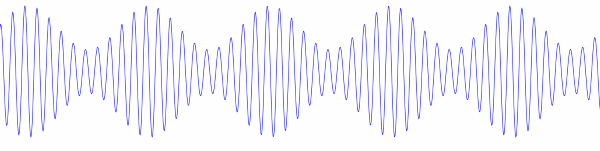In practice, however, the carrier is not multiplied by a signal like cos 2πbt but by 1 + m cos 2πbt where |m| < 1 to avoid over-modulation. Without this extra factor of 1 the signal would be 100% modulated; the envelope of the signal would pinch all the way down to zero. By including the factor of 1 and using a modulation index m less than 1, the signal looks more like the image above, with the envelope not pinching all the way down. (Over-modulation occurs when m > 1. Instead of the envelope pinching to zero, the upper and lower parts of the envelop cross.)